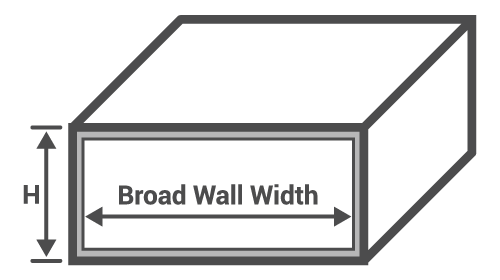# Waveguide Calculator (Rectangular)

## This tool calculates the cutoff frequency of a rectangular waveguide

GHz
GHz

### Overview

Microwave signals tend to radiate when they propagate down ordinary conductors. A waveguide is a transmission line that contains microwave signals inside a hollow tube and prevents them from radiating outward. It acts like a filter, allowing only microwave signal frequencies within a certain bandwidth. This tool is designed to calculate the center frequency of a rectangular waveguide and its bandwidth. Select the waveguide type and the broad wall width for that type will be generated automatically.### Equations

$$f_{c} = \frac{c}{2w_{m}}$$

$$f_{high} = 1.89f_{c}$$

$$f_{low} = 1.25f_{c}$$

Where:

$$f_{c}$$ = center frequency

$$w_{m}$$ = broad wall width of the waveguide in meters

$$f_{high} - f_{low}$$ = operating bandwidth

### Applications

Waveguides are transmission lines made from hollow tubes and commonly comes in rectangular or circular form. It only allows the propagation of microwave signals within a certain range of frequency. They hold the advantage over coaxial cable in terms of ease of manufacturing because of the absence of an inner conductor and shielding. As only air is found inside the waveguide, very high power signals can propagate safely through it without fear of dielectric breakdown. Radiation loss is also small if not zero in a waveguide compared to a coaxial cable. The coaxial cable however is lighter and less expensive compared to the waveguide. The bandwidth of signals inside a waveguide is also smaller compared to that on coaxial cables.